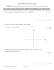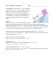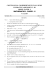# Sample Solutions to Quiz 3 for MATH3270A − y

## Transcription

Sample Solutions to Quiz 3 for MATH3270A − y
```Sample Solutions to Quiz 3 for MATH3270A
October 31,2013
1.Find the general solution of the ODE:
y (3) − y (2) + y 0 − Y = e−t sin t.
r3 − r2 + r − 1 = 0
The roots are r1 = 1,r2 = i,r3 = −i.The general solution of the homogeneous equation is:
yh = c1 et + c2 cos t + c3 sin t
A particular solution has the form yp = Ae−t sin t + Be−t cos t. Substitute it into the equation we get A = − 51 , B = 0. Thus the general
solution is
1
y = c1 et + c2 cos t + c3 sin t − e−t cos t
5
2.Show that W [1, sin2 t, cos 2t] ≡ 0 for all t.Can you prove this without
direct evaluation?
Answer: Since cos 2t = cos2 t − sin2 t = 1 − 2 sin2 t,thus 1,sin2 t,cos2 t
are linearly dependent,then Wronskian must be zero.
3.Find the Wronskian of a fundamental set of solutions to the ODE:
ty (3) + 2y (2) − 5y = 0, t > 0
1
2
By Abel’s formula:
Z
W (t) = c exp[−
2
dt] = c exp[−2 ln |t|] = ct−2
t
```

### Higher Relationships and Calculus NAB Revision Pack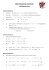### pdf file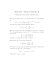### AS Entrance Examination Sample Paper Mathematics Instructions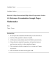### Math 120 Sample questions for Test 2, with answers### MAT 111 Exam 1 Fall 2014 Name:SOLUTIONS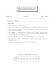### MATH 307: Problem Set #6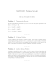### Calculus I Final, Sample### 1.6 Exam advice and sample questions for Chapter 1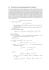### Test4-Review - FIU Faculty Websites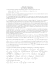### Time : 120 Minutes Max. Marks : 60 GENERAL INSTRUCTIONS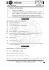### Sample Question Paper for Class 12 CBSE 2013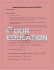### Homework 33: Stokes Theorem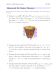### Math 121 Sample Problems for Test 3 e 1. lim### M427K (54315) Midterm #1 Solutions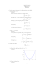### Worksheet 6 Chain rule and implicit differentiation 1 Chain rule 2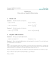### Document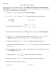### No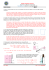### Chapter 6 Problems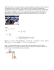### Written Homework 9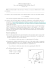### Departmental Practice Final Version 2 15400